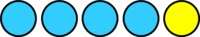# Fact Families with Pictures

Alignments to Content Standards: 1.OA.B

Write as many equations for each picture as you can.

1. Use the numbers 4, 1, and 5.Here are some equations for this picture. $$4+1=5 \hskip4em 5 = 4+1$$ $$5-1=4 \hskip4em 4=5-1$$ Can you find more equations?

2. Use the numbers 3, 5, and 8.3. Use the numbers 4, 4, and 8.4. Use the numbers 4, 5, and 9.5. Draw your own picture and write all the equations you can think of to describe it.

## IM Commentary

1. $$4+1=5 \hskip4em 5 = 4+1$$ $$1+4=5 \hskip4em 5 = 1+4$$ $$5-4=1 \hskip4em 1=5-4$$ $$5-1=4 \hskip4em 4=5-1$$
2. $$3+5=8 \hskip4em 8 = 3+5$$ $$5+3=8 \hskip4em 8 = 5+3$$ $$8-3=5 \hskip4em 5=8-3$$ $$8-5=3 \hskip4em 3=8-5$$
3. $$4+4=8 \hskip4em 8 = 4+4$$ $$8-4=4 \hskip4em 4=8-4$$
4. $$4+5=9 \hskip4em 9 = 4+5$$ $$5+4=9 \hskip4em 9 = 5+4$$ $$9-4=5 \hskip4em 5=9-4$$ $$9-5=4 \hskip4em 4=9-5$$